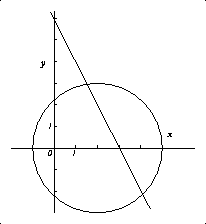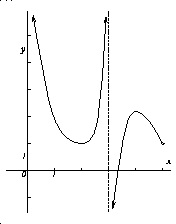Notation eFrog will be called exp(Frog) here, mostly because it is easier to type and read. Also, sqrt(Toad) will denote the square root of Toad (so sqrt(4) is 2).

Comment Answers will generally not be "simplified" unnecessarily. It is the philosophy of the management that formulas are delicate, and there is some risk of damaging them any time they are touched, so ... unless you really need to change them, don't bother.

Problem 1 B, the amplitude, is half of the difference between the maximum and the minimum, so B=2. The vertical shift A is the average of the maximum and minimum, so A= 7. Substituting x=0 will yield a value for C: algebraically y = A+Bsin(0+C) = 7+2sin(C). But (0,7.6) seems to be the y-intercept of the graph (at least approximately!) so that 7.6= 7+2sin(C). Solve this with your calculator (be sure to use radians!) and .3 will be the approximate value of arcsin(.3).

Problem 2 Arctan of a large number is close to Pi/2 (look at the graph of y = arctan x, and always use radians!). Pi is approximately 3.14, so arctan (1,000) is approximately 1.57, and 1,000 arctan (1,000) is 1570 (more or less) and the answer requested is 1600 (being careful to find the nearest 100!).

Problem 3 a) The functions ln and exp are inverses. Also, 3 ln (sqrt(x))= ln (x3/2), so exp(3 ln(sqrt(x)) ) = exp (ln (x3/2))= x3/2.
b) The standard properties of logs will be used. ln(B2 sqrt(A)) = 2 ln(B) + (1/2) ln A = 2 (5.3) + (1/2) (1.2)= 11.2. For the second part of the problem, we recall (look at the graph of ln!) that ln(Frog) < ln(Toad) exactly when Frog < Toad. Therefore we can compare A9 and B2 by comparing their lns. But ln(A9) = 9 ln(A)= 9 (1.2) =10.8 and ln(B2)=2 ln(B) = 2 (5.3) = 10.6, so that the first number is larger than the second.Problem 4 a) The equation of the circle is (x-2)2 + y2 = 9.
b)The equation of the line is y=-2x+6.
c) Maybe the easiest way is to substitute "y" from b) into the equation in a). This yields a quadratic in x which can be solved using the quadratic formula (important moral lesson: very few quadratics, even with small integer coefficients, can be "solved" by factoring!). The second coordinates can be gotten by using the equation in b). The two points gotten are ((14+sqrt(41))/5, (2-2sqrt(41))/5) and ((14-sqrt(41))/5, (2+2sqrt(41))/5). These points are approximately (1.52,-2.16) and (4.08,2.96). The picture may help some people understand the answers.Problem 5 a) The graph of y=Z(x) in the accompanying figure is the solid broken line.
b) Since 2 is in the interval [0,3], Z(2) should be evaluated using the first expression so Z(2) = 2*2 = 4. Since 4 belongs to the interval [3,infinity), we evaluate Z(4) using the second expression: Z(4) = 4+3=7.
c) Z is strictly increasing so it has an inverse N. For x in [0,3], Z(x)=2x, so the values of Z are in [0,6]. That means N(x) = (1/2)x for x in [0,6] (just solve y=2x for x!). Otherwise (for x > 6) N(x)= x-3 (gotten by solving y=x+3 for x). To find N(2) we use the first formula, so N(2)=(1/2)2=1, and N(8) uses the second formula, so N(8)=5.
d) The graph of y=N(x) in the accompanying figure is the dashed broken line.

Problem 6 The inverse function is given by n(p) = ((p-100)/3)2 and its domain coincides with the range of p(n) which is [103,130]. n(p) represents the number of boxes of detergent which can be produced for p dollars in this model.

Problem 7 a) The limit of the top is 0, and the limit of the bottom is 8 which is not 0. Therefore the limit of the expression is 0.
b) The bottom is x2 -4 = (x-2)(x+2), so if x is not equal to 2, the whole expression simplifies to 1/(x+2). The limit as x approaches 2 of this is just 1/4.
c) The bottom has a non-zero limit as x approaches 4, so we can get the limit just by evaluating the top and bottom at 4. The result is 0.
d) Multiply the top and bottom by sqrt(x)+2. Then the bottom becomes (sqrt(x)-2)(sqrt(x)+2) = x-4 which cancels the factor of (x-4) in the top and we're left with just sqrt(x)+2 on top. This has limit 4 as x approaches 4.

Problem 8 The derivative of f is f'(x) = 6x and f'(1)=6. So 6 is the slope of the line tangent to this curve when x=1. The tangent line has to pass through the point (1,f(1))=(1,7), so one equation for this line is y-7 = 6(x-1).

Problem 9In this problem, dx will denote the expression "delta x". Then f(x+dx)-f(x) = (3/(x+dx))-(3/x)= (3(x)-3(x+dx))/((x+dx)x)= (-3dx)/((x+dx)x). Therefore (f(x+dx)-f(x))/dx = -3/((x+dx)x). The limit of this last expression as dx approaches 0 is just -3/(x2).

Problem 10 a) (x3-3x+17)' = 3x2-3
b) (exp(x) sin(x))'= exp(x)' sin(x) + exp(x) (sin(x))' = exp(x) sin(x) + exp(x)cos(x).
c) ((2x+3)/(x2+1))' is a complicated fraction. For typographical purposes, think of it as Top/Bottom where Top= 2(x2 +1) -(2x+3)(2x) and Bottom = (x2+1)2. Please note: you are not asked to "simplify" the answer by any additional algebra.
d) (3exp(x) + (x/(2+5cos(x)) )' = 3exp(x) + another Top/Bottom where here Top= 1(2+5cos(x))-(0-5sin(x))x and Bottom= (2+5cos(x))2. Again, no additional "simplification" is needed.

Problem 11 By the chain rule the derivative of the left-hand side f'(g(x)) g'(x) which when x=2 is f'(g(2))g'(2)= f'(4)g'(2)= 2g'(2). The derivative of the right-hand side is 16x which when x=2 is 32. So we know that 2g'(2) = 32, and we get g'(2)=16.

Problem 12 a) tan(?) = 10/15 = 2/3, so ? = arctan(2/3) which is approximately 0.588 radians.
b) Here tan(alpha) = 10/A, so alpha = arctan(10/A).

Problem 13 a) If f is continuous at 0, the limit from the left should be equal to f(0). But the left-hand limit of f at 0 is equal to the left-hand limit of x2+2 at 0, and this limit is just 2. That means f(0) should be 2. We also know that f(0)=02 +A = A, and we know that the right-hand limit of f at 0 is the right-hand limit of the formula x2+A at 0, which is also A. Now the two limits and the value of f at 0 should agree to make f continuous at 0. This will occur when A=2.
b) The left-hand limit of f at 2 uses the formula x2 + 2 Here we substitute 2 for A, since 2 was the value found in the previous part of the problem. Therefore the left-hand limit of f at 2 is 22 +2 =6. The right-hand limit at 2 uses the formula x2+1, which has limit 5 at x=2. Since 5 is not equal to 6, f is not continuous at x=2.

Problem 14 At A the function is continuous (there are no jumps or breaks in the graph there) but it not differentiable. The graph has a "corner" at (A,g(A)): more formally, it looks like the right-hand part of the limit defining the derivative at A is 0 while the left-hand part of that limit is infinite (vertical lines have no slope). These don't agree, so the function is not differentiable at A.
The function is differentiable at B, and, since differentiable functions are continuous, it is also continuous at B.
The graph has a jump at x=C, so the function is not continuous there: the left- and right-hand limits differ (and neither agrees with the value of g at C. The function is not differentiable at C since if it were, it would have to be continuous there also.Problem 15 Vertical asymptotes are x=0 (the y-axis) and x=3. The accompanying graph satisfies the conditions given in the question. Note the "empty hole" at (5,1) since the function's domain does not include 5 (tricky!).

Problem 16 a) Consider the interval [2.5,3]. Enzyme concentration is increasing, since the slope of the tangent line is positive. But the rate of increase is "slowing" as time increases (moving from left to right along the curve) because the tangent line's slope is decreasing (such a curve is concave down).
b) Now consider the interval [1,2], for example. Here the enzyme concentration is also increasing because the slope of the tangent line is always positive. But in this interval as time increases the slope of the tangent line is increasing -- so the rate of increase is increasing here. This part of the curve is concave up. In both parts of this problem, the slope of the tangent line must be recognized as the rate of change of enzyme concentration. Also, there are other correct answers to this problem than the ones given here.

Problem 17 F(1) = f(1)g(1) = 2(5) = 10 and the product rule for derivatives gives the following: F'(1) = f'(1)g(1) + f(1)g'(1) = -1.
G(1) = f(1)/(g(1)+12) = 2/(5+1)= 2/6. The quotient rule shows that G'(x) = (f'(x)(g(x)+x2)-f(x)(g'(x)+2x))/((g(x)+x2)2) and evaluating this when x=1 yields G'(1)=-1.

Problem 18 a)(exp(3cos(2x)))'= exp(3cos(2x)) (3 cos(2x))' = exp(3cos(2x)) 3 (cos(2x))' = exp(3cos(2x))(3) (-sin(2x)) (2x)'= -6exp(3cos(2x))sin(2x)
b)(ln(x3+5x+9))'= (1/(x3+5x+9))(x3+5x+9)'= (1/(x3+5x+9) (3x2+5)
c) (sin((x+1)/(x2+2)))' = cos((x+1)/(x2+2)) ((x+1)/(x2+2))' = cos((x+1)/(x2+2)) (Top/Bottom) where here Top = (1)(x2+2) - 2x (x+1) and Bottom = (x2+2)2.